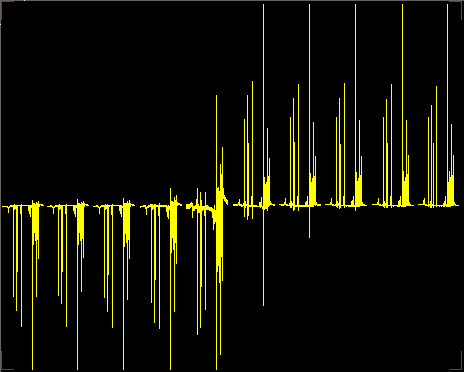Revision history[back]

# How to explain "binary" pattern in the nutation curve in a simple 90 degree pulse calibration experiment?

Hello, I wanted to ask this in a while. Finally a sample arrived that shows this behavior and I made a screenshot (below).

It's a 90 degree pulse calibration - using zero crossing near 360 degree pulse in standard one pulse FT experiment (arrayed pulse width at constant transmitter power).

Normally I expect a sine-wave-like picture as pulse width changes. Instead here there is a "binary" type of pattern - peak goes from all the way down then almost immediately all the way up. I've verified that there is no transmitter overflow - I've set receiver gain sufficiently low.

What's the physical explanation to this?# How to explain "binary" pattern in the nutation curve in a simple 90 degree pulse calibration experiment?

Hello, I wanted to ask this in a while. Finally a sample arrived that shows this behavior and I made a screenshot (below).

It's a 90 degree pulse calibration - using zero crossing near 360 degree pulse in standard one pulse FT experiment (arrayed pulse width at constant transmitter power).

Normally I expect a sine-wave-like picture as pulse width changes. Instead here there is a "binary" type of pattern - peak goes from all the way down then almost immediately all the way up. I've verified that there is no transmitter overflow - I've set receiver gain sufficiently low.low. Here step is at about 20o flip angle.

What's the physical explanation to this?# How to explain "binary" pattern in the nutation curve in a simple 90 degree pulse calibration experiment?

Hello, I wanted to ask this in a while. Finally a sample arrived that shows this behavior and I made a screenshot (below).

It's a 90 degree 90o pulse calibration - using zero crossing near 360 degree 360o pulse in standard one pulse FT experiment (arrayed pulse width at constant transmitter power).

Normally I expect a sine-wave-like picture as pulse width changes. Instead here there is a "binary" type of pattern - peak goes from all the way down then almost immediately all the way up. I've verified that there is no transmitter overflow - I've set receiver gain sufficiently low. Here step is at about 20o flip angle.

What's the physical explanation to this?# How to explain "binary" pattern in the nutation curve in a simple 90 degree pulse calibration experiment?

Hello, I wanted to ask this in a while. Finally a sample arrived that shows this behavior and I made a screenshot (below).

It's a 90o pulse calibration - using zero crossing near 360o pulse in standard one pulse FT experiment (arrayed pulse width at constant transmitter power).

Normally I expect a sine-wave-like picture as pulse width changes. Instead here there is a "binary" type of pattern - peak goes from all the way down then almost immediately all the way up. I've verified that there is no transmitter overflow - I've set receiver gain sufficiently low. Here step is at about 2010o flip angle.

What's the physical explanation to this?# How to explain "binary" binary-like pattern in the nutation curve in a simple 90 degree pulse calibration experiment?

Hello, I wanted to ask this in a while. Finally a sample arrived that shows this behavior and I made a screenshot (below).

It's a 90o pulse calibration - using zero crossing near 360o pulse in standard one pulse FT experiment (arrayed pulse width at constant transmitter power).

Normally I expect a sine-wave-like picture as pulse width changes. Instead here there is a "binary" type of pattern - peak goes from all the way down then almost immediately all the way up. I've verified that there is no transmitter overflow - I've set receiver gain sufficiently low. Here step is at about 10o flip angle.

What's the physical explanation to this?